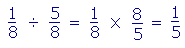S k i l l
i n
A R I T H M E T I C

Lesson 26  Section 2

# How to divide fractions

Section 1:  How to multiply fractions

WE ARE ABOUT to present an alternative to the method of  "Invert and multiply." (But see below.) It is based on a techniqe the student already knows, namely finding a common denominator.

For, in division, the dividend and divisor must be units of the same kind. They must have the same name. We can only divide dollars by dollars, hours by hours, yards by yards.

15 yards ÷ 3 yards = 5

-- because 5 times 3 yards = 15 yards. (Lesson 11.)

(We cannot divide 15 yards by 3 feet -- not until we change yards to feet.)

With fractions, the denominator names the units. (Lesson 21.) Therefore:

 67 ÷ 27 =  3.

"6 sevenths ÷ 2 sevenths = 3"

-- because 3 times 2 sevenths = 6 sevenths.

3 is how many times 2 sevenths are contained in 6 sevenths -- which is the answer to the question that division asks.

Here is the rule:

To divide fractions, the denominators must be the same.
The quotient will be the quotient of the numerators.

 Example 1. 1420 ÷ 1520 = 14  ÷ 15 = 1415 .

Example 2.   Prove:÷=.

Solution.   We must show that the quotienttimes the divisor,, will equal the dividend,. (Lesson 11.)

And on canceling the 15's --×=-- it does.  (Section 1, Example 9.)

Therefore when the denominators are the same, the quotient will be the quotient of the numerators.

 Example 3. 58 ÷ 78 = 57 .
 Example 4. 78 ÷ 58 = 75 =  1 25 .

Different denominators

When the denominators are not the same --

 58 ÷ 23

-- we can make a common denominator in the same way that we add fractions:

 58 ÷ 23 = 1524 ÷ 1624 = 1516 .

The common denominator here is  8 × 3 = 24.

 Example 5. 25 ÷ 34 = 8 20 ÷ 1520 = 8 15 .
 Example 6.    1 14 ÷  2 12 = 54 ÷ 52 = 54 ÷ 10 4 = 5 10 = 12 .

As in multiplication, we must change mixed numbers to improper fractions.  The common denominator is this example is 4.

 Example 7. 35 ÷  2 = 35 ÷ 10 5 = 3 10 .

To change a whole number into a fraction, multiply the whole number by the denominator.

 2  = 10 5

That product will be the numerator. (Lesson 21.)

Example 8.    A bottle of medicine contains 15 oz.  Each dose of the medicine is 2½ oz.  How many doses are there in the bottle?

Solution.   This is a division problem (Lesson 11) -- how many times can we subtract 2½ oz from 15 oz?

 15 ÷ 2½  =  15 ÷ 52 = 30 2 ÷ 52 =  30 ÷ 5 = 6.

In that bottle there are 6 doses.

Example 9.   On a map,of an inch represents 60 miles.  How many miles does 2 inches represent?

Solution.  For everyof an inch there are 60 miles.  How manyof an inch, then, are there in 2 inches?

 2 ÷ 34 = 84 ÷ 34 = 83 = 2 23 .

That number times 60 will produce the answer.

 83 × 60 = 8 × 20 = 160 miles.

Or:

 "Two and two thirds times 60 = Two times 60 + two thirds of 60 Lesson 16 = 120 + 40 = 160 miles."

For a solution based on proportions, see Lesson 23, Example 5.

"Invert and multiply"

A method often taught is to  "Invert the divisor and multiply."

 58 ÷ 23 = 58 × 32 = 1516 .

This gives the right answer, because equivalent to inverting, if we cross-multiplied --

 58 ÷ 23 = 15 ÷ 16 = 1516

-- we obtain the numerators if we changed those fractions to a common denominator.

When we invert a fraction, that number is called its reciprocal.  The

 reciprocal of 23 is 32 .

We can state the rule as follows:

Multiply by the reciprocal of the divisor.

 Example 9. 40 ÷ 45 = 40 × 54 = 10 × 5 = 50

Take the reciprocal of the divisor -- the number after the division sign ÷ .  Divide 4 into 40, then multiply.

Note that reciprocals come in pairs.

The reciprocal ofis, or 2.  And the reciprocal of 2 is.

In summary:

 5. How do we divide fractions?The denominators must be the same. The quotient will be the quotient of the numerators.To get the same answer: Take the reciprocal of the divisor, and multiply.Please "turn" the page and do some Problems.

or

Continue on to the next Lesson.

Previous Section